### Home > CALC > Chapter 5 > Lesson 5.2.5 > Problem5-104

5-104.
1. Let f(x) = x1/3. Homework Help ✎

1. Use the formal definition of continuity to determine if f(x) is continuous at x = 0.

2. Calculate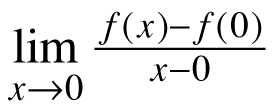.

3. What does the limit in part (b) represent?

4. What is happening graphically on f(x) at x = 0 that causes your answer to part(b)?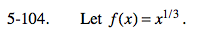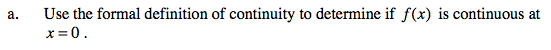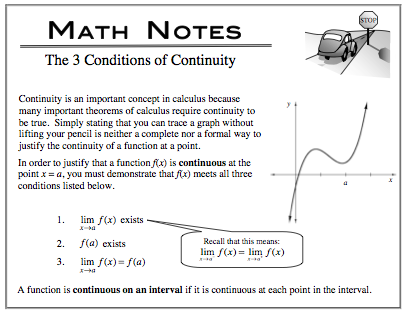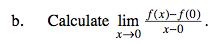This is Ana's definition of the derivative. The limit is equivalent to f '(0).So you can use the power rule to find the limit, if it exists.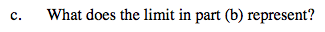Refer to the hint in part (b).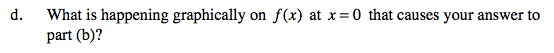Recall that derivatives do not exist at locations where f(x) has a: cusp, endpoint, jump, hole or vertical tangent.

Sketching the graph may help visualize the issue.